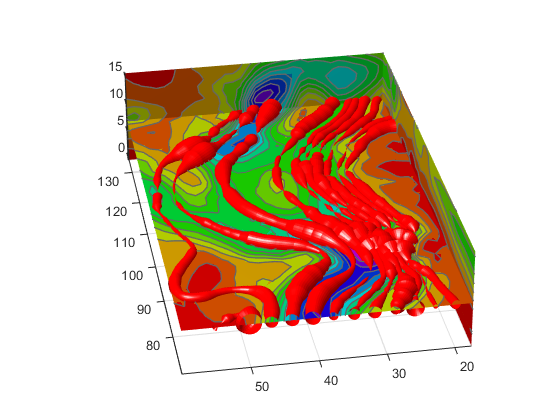## Displaying Divergence with Stream Tubes

### What Stream Tubes Can Show

Stream tubes are similar to stream lines, except the tubes have width, providing another dimension that you can use to represent information.

By default, MATLAB® graphics display the divergence of the vector field by the width of the tube. You can also define widths for each tube vertex and thereby map other data to width.

This example uses the following techniques:

• Stream tubes to indicate flow direction and divergence of the vector field in the `wind` data set

• Slice planes colored to indicate the speed of the wind currents overlaid with contour line to enhance visibility

Inputs include the coordinates of the volume, vector field components, and starting locations for the stream tubes.

### 1. Load Data and Calculate Required Values

Load the data and calculate values needed to make the plots. These values include:

• The location of the slice planes (maximum `x`, minimum `y`, and a value for the altitude)

• The minimum `x` value for the start of the stream tubes

• The speed of the wind (magnitude of the vector field)

```load wind xmin = min(x(:)); xmax = max(x(:)); ymin = min(y(:)); alt = 7.356; % z value for slice and streamtube plane wind_speed = sqrt(u.^2 + v.^2 + w.^2); ```

### 2. Draw the Slice Planes

Draw the slice planes (`slice`) and set `surface` properties to create a smoothly colored slice. Use 16 colors from the `hsv` `colormap`.

```hslice = slice(x,y,z,wind_speed,xmax,ymin,alt); set(hslice,'FaceColor','interp','EdgeColor','none') colormap hsv(16) ```

### 3. Add Contour Lines to Slice Planes

Add contour lines (`contourslice`) to the slice planes. Adjust the contour interval so the lines match the color boundaries in the slice planes:

• Call `caxis` to get the current color limits.

• Set the interpolation method used by `contourslice` to `linear` to match the default used by `slice`.

```color_lim = caxis; cont_intervals = linspace(color_lim(1),color_lim(2),17); hcont = contourslice(x,y,z,wind_speed,xmax,ymin,... alt,cont_intervals,'linear'); set(hcont,'EdgeColor',[.4 .4 .4],'LineWidth',1) ```

### 4. Create the Stream Tubes

Use `meshgrid` to create arrays for the starting points for the stream tubes, which begin at the minimum `x` value, range from 20 to 50 in `y`, and lie in a single plane in `z` (corresponding to one of the slice planes).

The stream tubes (`streamtube`) are drawn at the specified locations and scaled to be 1.25 times the default width to emphasize the variation in divergence (width). The second element in the vector [1.25 30] specifies the number of points along the circumference of the tube (the default is 20). You might want to increase this value as the tube size increases, to maintain a smooth-looking tube.

Set the data aspect ratio (`daspect`) before calling `streamtube`.

Stream tubes are surface objects, therefore you can control their appearance by setting surface properties. This example sets surface properties to give a brightly lit, red surface.

```[sx,sy,sz] = meshgrid(xmin,20:3:50,alt); daspect([1,1,1]) % set DAR before calling streamtube htubes = streamtube(x,y,z,u,v,w,sx,sy,sz,[1.25 30]); set(htubes,'EdgeColor','none','FaceColor','r',... 'AmbientStrength',.5) ```

### 5. Define the View

Define the view and add lighting (`view`, `axis` `volumebounds`, `Projection`, `camlight`).

```view(-100,30) axis(volumebounds(x,y,z,wind_speed)) set(gca,'Projection','perspective') camlight left ```